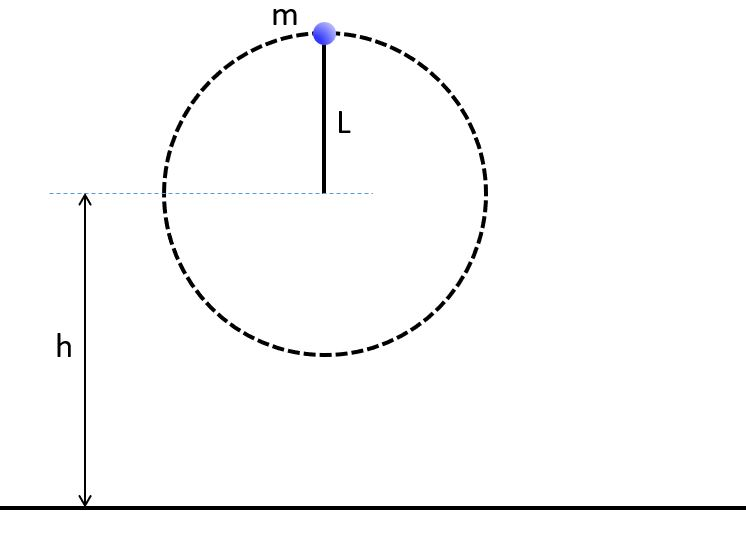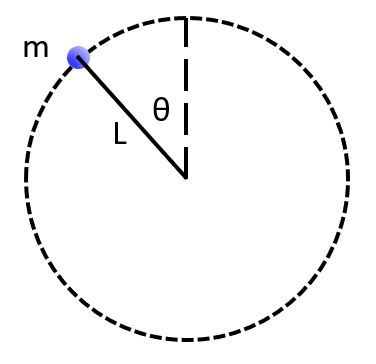Circular motion applications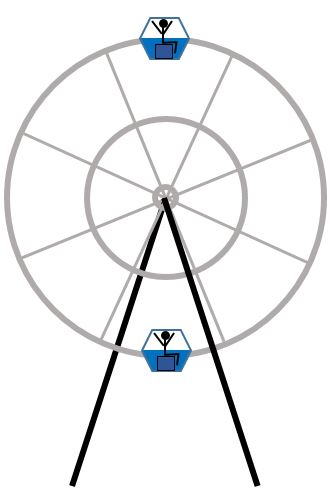1. A person rides on a Ferris wheel of radius r at constant speed v. (Image is exaggerated. Assume the person is on the rim of the wheel.)

How does the normal force exerted on the rider at the top compare to the normal force on the rider at the bottom?

A. Ntop > Nbottom

B. Ntop = Nbottom

C. Ntop < Nbottom

2. An out-of-gas car is rolling over the top of a hill at speed v. At this instant, which statement is true?

A. N > Fg

B. N = Fg

C. Fg > N

3. Consider a car of mass m going around a curve of radius r on level ground at a constant speed v.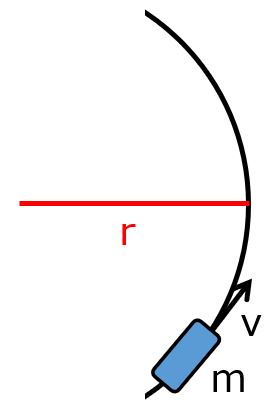What assumptions can you make about the system?

X. There is no air resistance.
Y. There is no friction.

A. Only X is a valid assumption.

B. Only Y is a valid assumption.

C. Both X and Y are valid assumptions.

D. Neither X nor Y is a valid assumption.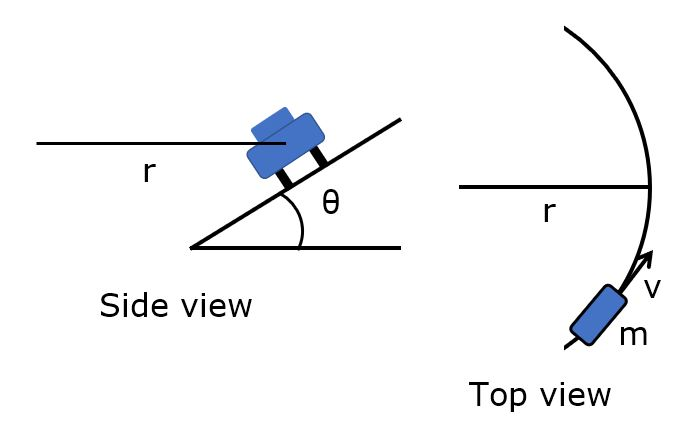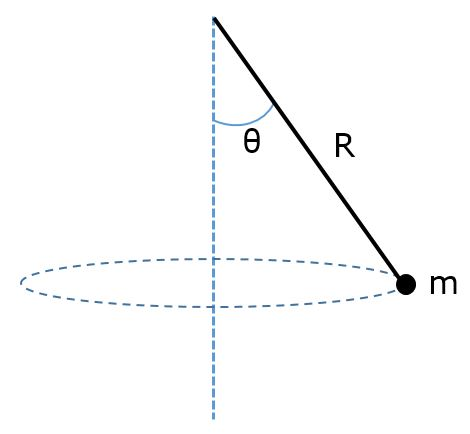7. A ball of mass m is tied to the end of a string of length L and swung in a vertical clockwise circle. The center of the circle is a distance h above the floor. The ball is swung at the minimum speed necessary to make it over the top without the string going slack. If the string is released at the moment the ball is at the top of the circle, how far to the right does the ball hit the floor?